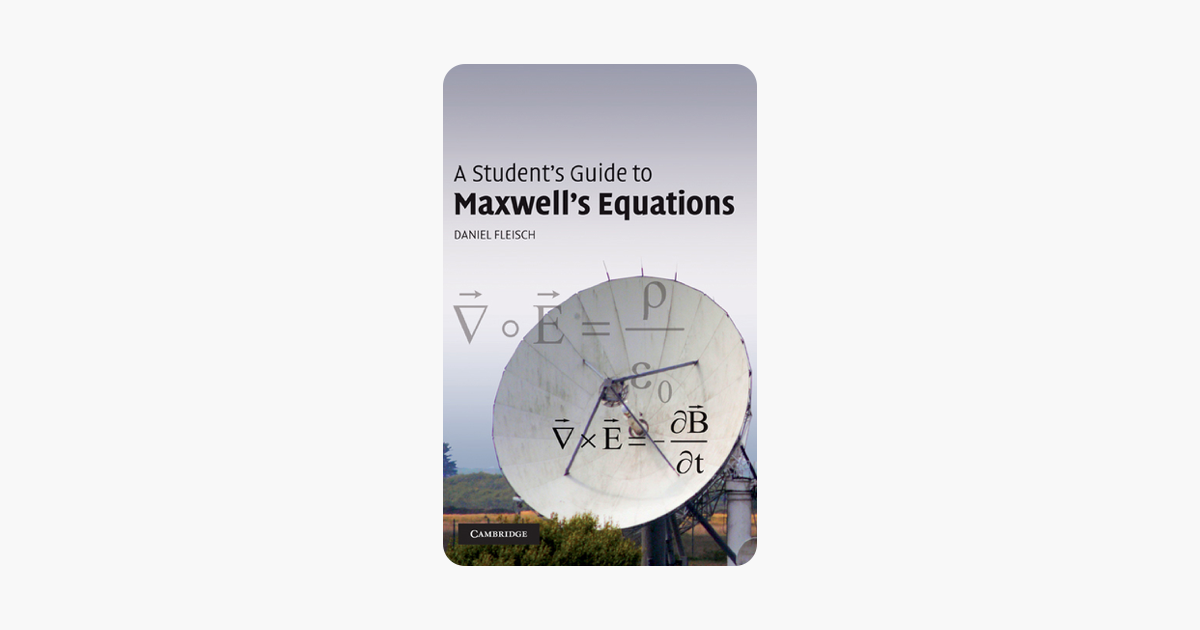# A Student's Guide to Maxwell's Equations - Daniel Fleisch

93 of 767Gauss's law for electric fields, Gauss's law for magnetic fields, Faraday's law, and the Ampere–Maxwell law are four of the most influential equations in science. In this guide for students, each equation is the subject of an entire chapter, with detailed, plain-language explanations of the physical meaning of each symbol in the equation, for both the integral and differential forms. The final chapter shows how Maxwell's equations may be combined to produce the wave equation, the basis for the electromagnetic theory of light. This book is a wonderful resource for undergraduate and graduate courses in electromagnetism and electromagnetics. A website hosted by the author at www.cambridge.org/9780521701471 contains interactive solutions to every problem in the text as well as audio podcasts to walk students through each chapter.

CLOSE [x]